# MHD Nanoliquid Flow Along a Stretched Surface with Thermal Radiation and Chemical Reaction Effects

MHD Nanoliquid Flow Along a Stretched Surface with Thermal Radiation and Chemical Reaction Effects
Department of Mathematics, College of Engineering and Technology, SRM Institute of Science and Technology, Kattankulathur–603203, Tamil Nadu, India

Corresponding Author Email:
vennilab@srmist.edu.in
Page:
1704-1710
|
DOI:
https://doi.org/10.18280/mmep.090632
16 September 2022
|
Accepted:
19 December 2022
|
Published:
31 December 2022
| Citation

OPEN ACCESS

Abstract:

The consequences of viscous, ohmic, thermal radiation, and chemical reaction on an incompressible, steady, magnetized nanofluid moving through a stretched surface are investigated for choosing two distinct Nanoliquids (Cuo/water and Silver/water). Through the similarity transformations, the controlling dimensional equations of momentum, energy, and concentration were simplified to non-dimensional forms. The solution to the resulting problem is obtained by Bvp4c. The velocity, temperature and concentration are significantly shown for various emerging factors. On both kinds of nanofluids, thermal is grown consistently with an increasing Eckart number. It does, however, lessen as the radiation term values increase. Moreover, if the Schmidt number ranges on the concentration fluid flow indicate that the H(η) profile is going to fall. The tendency for H(η) to boost as the Soret number raises is becoming increasingly noticeable. Ag/water has a higher heat flow rate than Cuo/water when the amounts of Eckart and Radiation numbers are changed. Tables and plots displayed an engineering physical parameter such as coefficient of skin friction, rate of heat transmission and mass transfer.

Keywords:

magneticfield, eckart number, thermal radiation, schmidt number, soret number

1. Introduction

Nanofluids covers a comprehensive variety of technology improvements, such as industry cooling systems, nanofluid cooler, geothermal power extraction, nuclear reactors, electronic circuit cooling, combustion, and so on . Nanofluid is a dispersion of Nanoparticles in standard fluids(kerosine, ethylene glycol, water). It is dramatically improve their performance at industrial processes and scientific circles. Metals, oxides, and carbon nanotubes are commonly used to produce NP’s. The word Nanofluid was introduced by Choi  in the year 1995. The research of smooth flow of thermal convection over a stretching/shrinking sheet is a significant concern due to its growing engineering settings and relevance in a wide range of technical operation. Many engineering fields deals with boundary layer flow along a stretching surface. Aleem et al.  illustrated the effects of heat generating, chemical reacting, and radiation of five nano particles with a normal fluid water flow across a vertical plate. The laplace transformation is used to resolve this fluid problem. Anjali Devi and Prakash  used Runge kutta integration technique to resolve the laminar stream through a stretched space with thickness and power law velocity in the occurence of megnetic field. Kandasamy and Devi  studied the impact of heat and mass transmission on the occurrence of chemical changes along with the humid object. Furthermore, numerous researchers have used computational and analytical tools to examine the impact of radiation, and thermal expansion over a stretching sheet. Because of their essential physical attributes. Hazarika et al.  expressed the Magneto hydrodynamic movement of heat production, chemically reacting nanomaterials/H2O over an elongating penetrable medium and finding the solution of non linear ODE problem by RK-Shooting techniques. Afridi and Qasim  presented the entropy production of Cu-H2O and Ag-H2O two nanofluids moved along a slendring stretching space. Esmawan et al.  explored the Cuo-H2O nanofluid can be utilized as a cooling medium due to its high thermal conductivity. Yirga and Tesfay  studied the behavior of viscous and chemical reaction on heatflux of magnetic nanoliquid stream over a porose space. Ghasemia and Hatamib  explored the sun ray effects on two-dimensional stagnation point flow of magnetic nanofluid along a stretching surfce. Ganga et al. , Khidir and Sibanda  and Hamad and Ferdows  studied the consequences of thermophoresis, thermal absorption/generation, brownian motion of nanofluid as a fuction of surface.

The function of an oblique magnetic field on Casson nanofluid over a stretched sheet contained in a wet pore spaces with the condition of radiation and a uneven heat sink/source is analyzed by Panigrahi et al. . Thiagarajan and Dinesh  examined the chemically reacting heat flux and hydromagnetic nanofluid stream through a exponentially stretching surface with viscous, radiation and ohmic effects. Babu et al.  investigated a pssive heat exchanger and radiating Mhd non newtonian nanoliquid due to extending surface. Haq et al.  analyzed the effects of aligned magnetic field of CNTs in two distinict standard fluids along a moved slip plane. Satya Narayana et al.  studied the numerical result of non linear radiating on the three dimensional casson nanofluid of a double stress surface. Sithole et al.  addressed the Mhd, viscous, non linear radiation and genertation of entropy in second grade nanofluid moves through a stretching surface. Khan et al.  incorporated the impacts of radiation, Mhd, multiple slip on viscoelastic nanofluid’s unsteady stream over a penetrable medium. Ridha and Al-Abboodi  inspected the two phase liquid-solid hydordynamics of inclined fluidized beds. Piancastelli  revealed the impact of outside warm air recycling to provide continuous, high efficiency defrosting of air-to-air heat pumps.

The idea of this paper were srcuntized by Shankar and Gorfie  to implenting the comparative analysis are done between two naoliquids such as copper oxide/water and silver/water with the impacts of magnetic,electrical,thermal radiation,chemical reaction. The physical quantaties Skin friction, Nusselt and sherwood numbers are have been explored through Graphs and Tabels(II - V). The thermophysical attributes of copper oxide, water and silver are given in Table 1 is refered from [7, 24].

Table 1. Thermophysical characteristics

 Fluid and Nanoparticles Water Cuo Ag Density ρ (kg/m3) 997.1 6500 10500 Thermal condunctivity K(w/mk) 0.613 18 429 Thermal capacity Cp 4179 540 235
2. Flow Model

Assumed that in a stretching surface, the steady boundary layer flow of electrical and magnetic nanofluid moves on it. Suppose a system of cartesian coordinate under which the surface relates to the x and y axis and the liquid occupies the designated space y≥0. Taken u=Uw(x) characterizes surface velocity in the region of x. The axis y is subjected to a homogeneous vertical magnetic field of strength B0(x). The generated magnetic field, external electric field and electric field related to polarized changes are supposed to be insignificant compared to the used magnetic field (Figure 1). The flow is created by the constant extending of the surface, which is achieved by the operation of two equivalent and opposing forces on the axis-x to hold the starting point is stationary. Let $T_w$ and $C_w$ are surface temperature and concentration respectively. The liquid has a systematic ambient temperature, concentration as $T_{\infty}, \ C_{\infty}$ where $T_w>T_{\infty}, C_w>C_{\infty}$. The following are the equations for continuity, momentum, energy and nanomaterials fraction [23, 25, 26].

## 1.jpg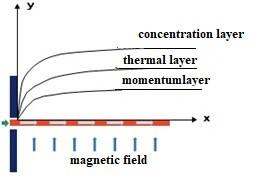Figure 1. Physical depiction of flow model

$u \frac{\partial u}{\partial x}+v \frac{\partial v}{\partial y}=0$    (1)

$u \frac{\partial u}{\partial x}+v \frac{\partial u}{\partial y}=\frac{\mu_{n f}}{\rho_{n f}} \frac{\partial^2 u}{\partial y^2}-\frac{\sigma B_0^2(x) u}{\rho_{n f}}$    (2)

\begin{aligned} u \frac{\partial T}{\partial x}+v \frac{\partial T}{\partial y} & =\alpha_{n f} \frac{\partial^2 T}{\partial y^2}+\frac{\mu_{n f}}{\left(\rho C_p\right)_{n f}}\left(\frac{\partial u}{\partial y}\right)^2 \\ & -\frac{1}{\left(\rho C_p\right)_{n f}} \frac{\partial q_r}{\partial y}+\frac{\sigma B^2(x) u^2}{\left(\rho C_p\right)_{n f}}\end{aligned}    (3)

$u \frac{\partial C}{\partial x}+v \frac{\partial C}{\partial y}=D\left(\frac{\partial^2 C}{\partial y^2}\right)+D_1\left(\frac{\partial^2 T}{\partial y^2}\right)-K_0\left(C-C_{\infty}\right)$    (4)

$v=0, \quad u=b_1 x, \quad T=T_W, C=C_W a t \ y=0$     (5)

$u \rightarrow 0, T \rightarrow T_{\infty,\quad} C \rightarrow C_{\infty}$ at $y \rightarrow \infty$    (6)

where, $\left(\rho C_p\right)_{n f}, \ \mu_{n f}, \rho_{n f}, \ \alpha_{n f}$ are represented as heat capacitance, dynamic viscosity, effective density and thermal diffusivity of nanoliquid are given below:

$\mu_{n f}=\frac{\mu_f}{(1-\varphi)^{5 / 2}}$

$\rho_{n f}=(1-\varphi) \rho_f+\varphi \rho_f$

$\alpha_{n f}=\frac{k_{n f}}{\left(\rho C_p\right)_{n f}}$

$\left(\rho C_p\right)_{n f}=\varphi\left(\rho C_p\right)_s+(1-\varphi)\left(\rho C_p\right)_f$    (7)

Maxwell-Granett model approximate the thermal conductivity of nanofluids confined to spherical nano particle.

$\frac{k_{n f}}{k f}=\frac{k_f-2 \varphi\left(k_f-k_s\right)+2 k_f}{k_s+\varphi\left(k_f-k_s\right)+2 k_f}$

Let us introduce a similarity transformations:

$\left.\begin{array}{c}u=b_1 x, v=-\left(b_1 v_f\right)^{0.5} \\ T=G(\eta)\left(T_w-T_{\infty}\right)+T_{\infty} \\ C=H(\eta)\left(C_w-C_{\infty}\right)+C_{\infty} \\ \eta=y\left(b_1 / v_f\right)^{0.5} \\ \psi=\left(b_1 v_f\right)^{0.5} x F(\eta)\end{array}\right\}$   (8)

Incorporating the above mentioned transformation into the governing Eqns. (1) to (4) reduces to:

$\mathrm{F}^{\prime \prime \prime}+\mathrm{S}_1 F F^{\prime \prime}-\mathrm{F}^{\prime}\left(\mathrm{S}_1 \mathrm{~F}^{\prime}+\mathrm{S}_1 \mathrm{~S}_2^{-1} \mathrm{M}\right)=0$    (9)

$\left(\frac{\mathrm{k}_{\mathrm{nf}}}{\mathrm{k}_{\mathrm{f}}}+\mathrm{NR}\right) \mathrm{G}^{\prime}+\mathrm{PrS}_3\left(\mathrm{FG}^{\prime}-2 \mathrm{~F}^{\prime} \mathrm{G}\right)+\operatorname{EcPr}\left(\mathrm{S}_3 \mathrm{~S}_4^{-1} \mathrm{~F}^{\prime \prime 2}+\mathrm{MF}^2\right)=0$     (10)

$\mathrm{H}^{\prime \prime}-\mathrm{Sc}\left(2 \mathrm{HF}^{\prime}-\mathrm{F} H^{\prime}+\mathrm{G} \gamma\right)=0$    (11)

and the corresponding boundary conditions are:

$\mathrm{F}(0)=0, F^{\prime}(0)=1, \mathrm{G}(0)=1, \mathrm{H}(0)=1$ at $\eta=0$    (12)

$F^{\prime}(\eta) \rightarrow 0, \mathrm{G}(\eta) \rightarrow 0, \quad \mathrm{H}(\eta) \rightarrow 0$ at $\eta \rightarrow \infty$    (13)

where, the dimesional less parameters are:

$\operatorname{Pr}=\frac{v}{\alpha}, M=\frac{\sigma B_0{ }^2}{b_1 \rho_f}, E c=\frac{U_w{ }^2}{\left(C_p\right)_f\left(T_w-T_{\infty}\right)}$

$N R=\frac{16 \sigma^* T_{\infty}^2}{3 k_1 k_f}, \gamma=K_0 / b_1, S c=\frac{v}{D}, S r=\frac{D_1\left(T_w-T_{\infty}\right)}{D\left(C_w-C_{\infty}\right)}$

$S_1=(1-\varphi)^{5 / 2}\left[1-\varphi+\varphi\left(\frac{\rho_s}{\rho_f}\right)\right]$

$S_2=\left[1-\varphi+\varphi\left(\frac{\rho_s}{\rho_f}\right)\right], S_3=1-\varphi+\varphi \frac{\left(\rho C_p\right)_s}{\left(\rho C_p\right)_f}$

$S_4=(1-\varphi)^{5 / 2}\left[1-\varphi+\varphi \frac{\left(\rho C_p\right)_s}{\left(\rho C_p\right)_f}\right]$

Wall friction $C_f$, Local Nusselt number $N u_x$, Sherwood number $S h_x:-$

In the heat transfer issue, the physical quantaties are very important

$C_f(1-\varphi)^{2.5} \sqrt{R e_x}=-2 F^{\prime \prime}(0)$    (14)

$\left(N u_x / \sqrt{R e_x}\right)\left(k_f / k_{n f}\right)=-\left(\frac{k_{n f}}{k_f}+N R\right) G^{\prime}(0)$    (15)

$\left(\begin{array}{l}S h_x / \sqrt{R e_x}\end{array}\right)=-H^{\prime}(0)$    (16)

where, the local Reynolds number is $R e_x=x u_w / v_f$.

3. Graphical Representation

## 2.png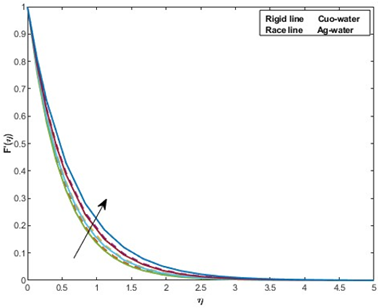Figure 2. Behavior of φ=0.1,0.2,0.3,0.4 on F'(η)

## 3.png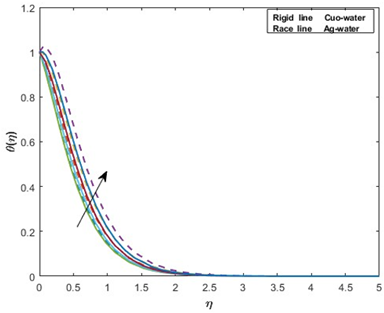Figure 3. Behavior of φ=0.1,0.2,0.3,0.4 on G(η)

## 4.png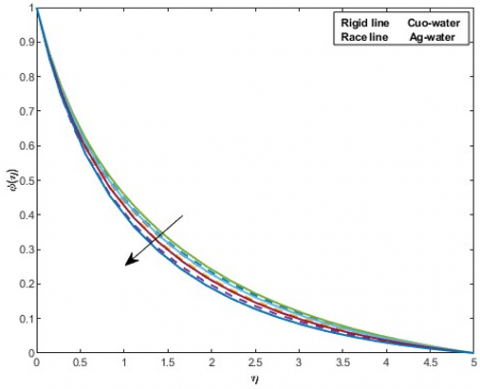Figure 4. Behavior of φ=0.1,0.2,0.3,0.4 on H(η)

## 5.png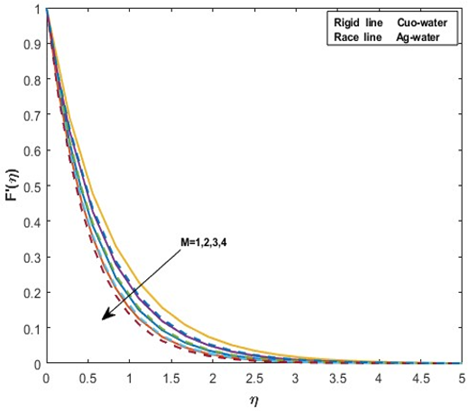Figure 5. Behavior of M on F'(η)

## 6.png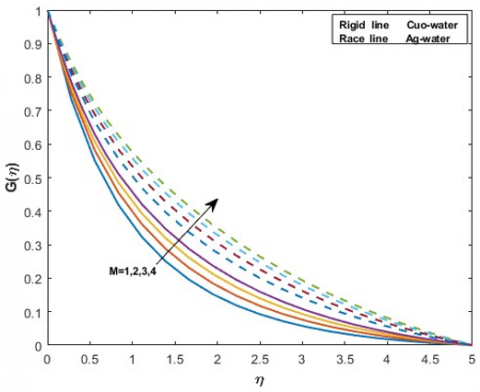Figure 6. Behavior of M on G(η)

## 7.png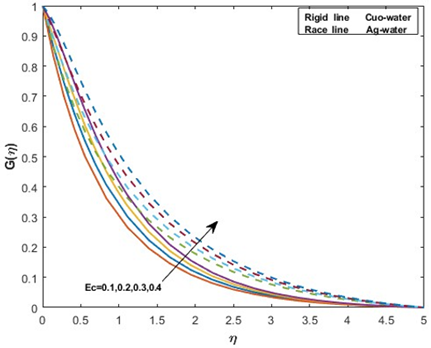Figure 7. Behavior of Ec on G(η)

## 8.png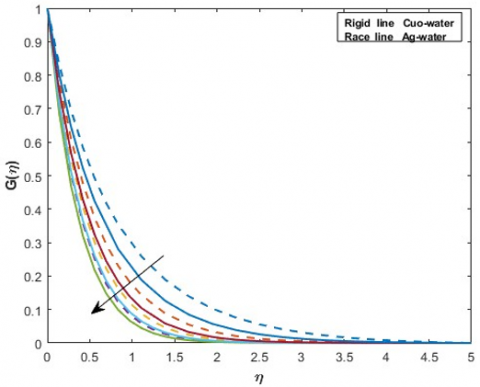Figure 8. Behavior of Nr = 0.3,0.5,0.7,0.9 on G(η)

## 9.png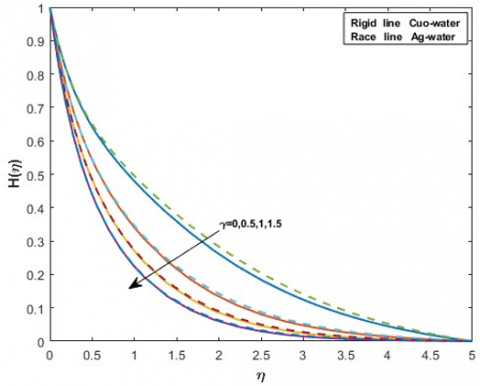Figure 9. Behavior of γ on H(η)

## 10.png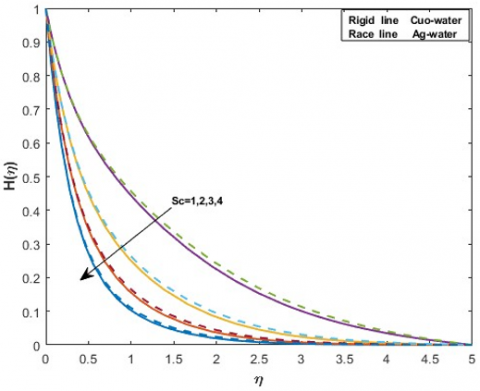Figure 10. Behavior of Sc on H(η)

## 11.png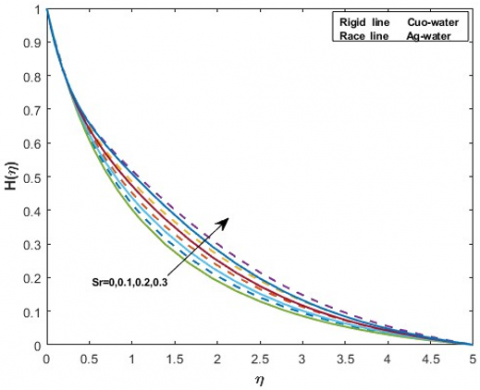Figure 11. Behavior of Sr on H(η)

## 12.png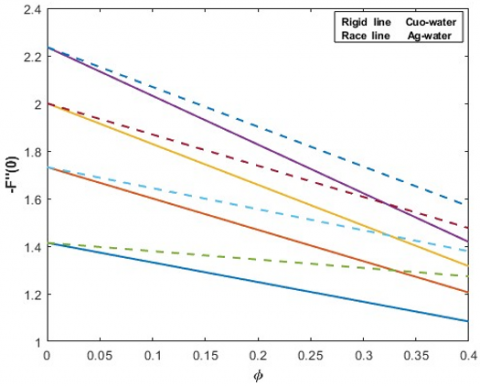Figure 12. Behavior of -F''(0)

## 13.png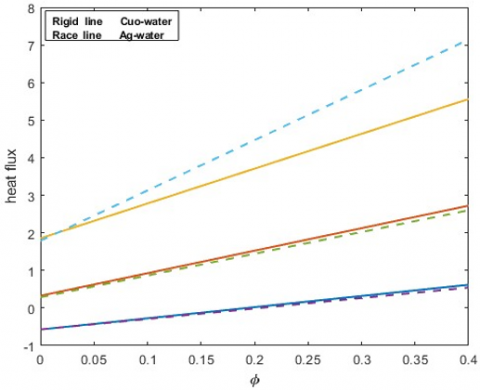Figure 13. Behavior of heat flux

## 14.png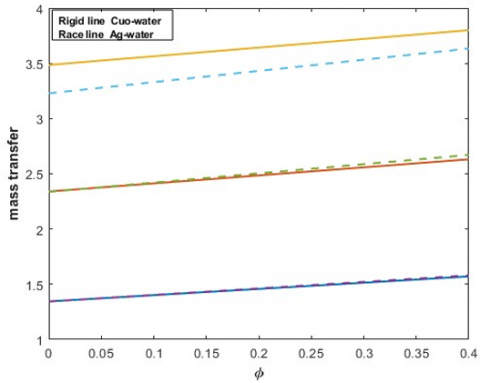Figure 14. Behavior of mass transfer

4. Result and Discussion

Employing Bvp4c, the issue of steady incompressible magnetic nanofluid exposed to the interaction of radiation and chemical reaction are resolved.

Table 2. Comparison of the value of wall temperature gradient -G’(0) for different values of prandtl numbers Pr

 Pr 0.72 1 3 10 100 Kameswaran27 1.08852 1.33333 2.50973 4.79687 15.7116 Bandari & Eshetu23 1.08862 1.33333 2.50973 4.797 15.7198 Present study 1.08807 1.33651 2.51041 4.79075 15.7064

Table 3. Skin friction

 Silver/water φ M=1 M=2 M=3 M=4 0 0.1 0.2 0.3 1.414237 1.506424 1.495368 1.411504 1.732051 1.742890 1.675841 1.549874 2.000000 1.950921 1.838710 1.676909 2.236067 2.138814 1.988287 1.794985 Copper oxide/water φ M=1 M=2 M=3 M=4 0 0.1 0.2 0.3 1.414237 1.400385 1.333021 1.224412 1.732051 1.652090 1.532702 1.381582 2.000000 1.870247 1.709263 1.522710 2.236067 2.065492 1.869226 1.651844

Figures 2, 3 and 4 are exhibits an interaction of volume fraction on momentum, thermal and concentration distributions. In these plots, varying the values of φ accelerates the momentum and heat boundary layers. But we have the opposite result on the concentration boundary layer. The magnetic variable M on energy and velocity distributions are depicted on Figures 5 and 6. It can be seen that as the magnetic interaction parameter M increases, so does the temperature. Electrically conducting nanofluids have the lowest resistance due to the Lorentz force, so the thickness of the thermal layer is increased. It reveals that Ag/water nanofluid is faster than Cuo/water. But the same parameters of M decelerates the velocity profile. Figure 7 shows the Ec (viscous dissipation) influenced on the temperature field. By increasing Ec, greatest force is used to dispel the viscosity. From this we can observe that the temperature trend are rising as the Eckart number rises. Therefore the silver/water nanofluid increased faster than Cuo/water nanofluid in G(η) trend.

The behaviour of thermal radiation in the temperature field is predicted on Figure 8, it is depicted that the temperature field is diminished as the distinct values of NR number rises. In that sense, copper oxide nanofluid is decreased faster than silver nanofluid. Figure 9 illustrates that the chemical reaction influences H(η) in the stretched sheet. An Increment of the $\gamma$ on Ag/water concentration stream flows are reduced slower than Cuo/water in H(η). The effect of Sc number over concentration demonstrated on Figure 10. The higher value of Sc on concentration fluid flow, shows that decreasing trend for H(η). Here also, Ag/water flows are diminished slower than Cuo/water. Figure 11 illustrates the increasing trend of H(η) when rising value of Sr number. In this profile Cuo/water flows are greater than Ag/water liquid on H(η).

Table 4. Heat transfer

 φ M Ec NR Cuo/H2O Ag/H2O 0 3.5 0.4 0.5 -0.572471 -0.571118 0.1 -0.354474 -0.253104 0.2 -0.094855 0.111102 0.3 0.222061 0.543737 0.4 0.617706 1.075997 0 3.5 0.5 1 0.326598 0.285563 0.1 0.786823 0.942975 0.2 1.323770 1.702583 0.3 1.959464 2.600567 0.4 2.722927 3.687149 0 3.5 0.6 1.5 1.854260 1.788512 0.1 2.582843 2.830585 0.2 3.423554 4.033851 0.3 4.404306 5.448278 0.4 5.560916 7.142247

Table 5. Mass transfer

 φ M Ec NR γ Sc Sr Cuo/H2O Ag/H2O 0 3.5 0.4 0.5 0.5 1 0.1 1.344603 1.343643 0.1 1.386628 1.382557 0.2 1.437663 1.434346 0.3 1.498642 1.499700 0.4 1.570620 1.580097 0 3.5 0.4 0.5 1 2 0.2 2.339931 2.336706 0.1 2.396334 2.397088 0.2 2.462938 2.472098 0.3 2.540798 2.562742 0.4 2.631175 2.670813 0 3.5 0.4 0.5 1.5 3 0.3 3.485571 3.228511 0.1 3.548078 3.305495 0.2 3.620515 3.397781 0.3 3.704035 3.506679 0.4 3.799989 3.634224

Figures 12, 13 and 14 examine the skin friction, heat and mass transfers of velocity, thermal and concentration profiles against volume fraction. The skin friction rate of both nanofluids are decremented against φ = 0,0.1,0.2,0.3,0.4 and M = 1,2,3,4. Here, the Nanofluid Cuo/water have a lower friction rate comparing to Ag/water. The rate of heat is transferred for both nanofluids, when raising the parameters of M = 3.5, Ec = 0.4, 0.5,0.6 and NR = 0.5,1.0,1.5 Ag/water nanofluid have a greater heat transfer values than Cuo/water. In Figure 14, the first set of data demonstrates the monotonic behaviour of two different nanoliquids with respect to the M = 3.5, Ec = 0.4, Nr = 0.5, Sc = 1,2,3, Sr = 0.1,0.2,0.3 and $\gamma$= 0.5,1.0,1.5 parameters when plotted against the volume fraction.The second group of data, which exhibits the separate parameters Sc, Sr, and γ versus the volume fraction, shows that the ratio of Ag/water flows greater than the ratio of Cuo/water. But in the third category values are illutstrated that cuo/water flows faster than Ag/water. Overall, while rasisng respective parameters against the volume percent its shows that increment on mass transfer profile.

We made comparisons with earlier published article by Kameswaran  and Shankar and Gorfie  in the absence of thermal radiation, magnetic field, Ohmic heating to validate the accuracy of the numerical results. The results are in good agreement, as shown in Table 2. Table 3 shows the solution of skin friction for various volume fraction and magnetic field. Table 4 represents the values of heat flux of different volume fraction, Eckart and radiation parameters in the occurrence of M. Table 5 represents the values of mass transmision of different volume fraction, Soret number, Schmidt number and $\gamma$ in the occurrence of M, Ec, NR.

5. Conclusion

The issue of boundary layer flow of electrically, magnetized and chemically reacted nanoliquids(Ag/water and Cuo/water) along a stretched surface, with influence of radiation, viscous and ohmic are discussed. The nonlinear ODE solved by Bvp4c(matlab).

The result of the problem is:

(i) Thermal profile is constantly increased with increasing Eckart number on both kind of nanofluids. However, it is decreased with increasing radiation term values.

(ii) The higher values of Sc number on concentration fluid flow, shows that decreasing trend of H(η) profile. The increasing trend of H(η) occurs when raising value of Sr number.

(iii) By rising the levels of Eckart and radiation numbers, Ag/water having a greatest heat flux rate than Cuo/water.

Nomenclature
 Symbol Quantity ρs Density of nanoparticle ϕ Volume fraction ρf Density of base fluid (ρCp)s Heat capacity of nanoparicle (ρCp)f Heat capcity of base fluid knf Thermal conductivity of nanofluid ks Thermal conductivity of nanoparticle kf Thermal conductivity of base fluid Pr Prandtl number M Magnetic parameter Sc Schmidt number Ec Eckart number Sr Soret number NR Radiation parameter γ Chemical reaction parameter σ Electrical conductivity
References

 Wong, K.V., De Leon, O. (2010). Applications of nanofluids: current and future. Advances in mechanical engineering, 2: 519659. https://doi.org/10.115/2020/519659

 Choi, S.U., Eastman, J.A. (1995). Enhancing thermal conductivity of fluids with nanoparticles (No. ANL/MSD/CP-84938; CONF-951135-29). Argonne National Lab.(ANL), Argonne, IL (United States).

 Aleem, M., Asjad, M.I., Shaheen, A., Khan, I. (2020). MHD Influence on different water based nanofluids (TiO2, Al2O3, CuO) in porous medium with chemical reaction and newtonian heating. Chaos, Solitons & Fractals, 130: 109437. https://doi.org/10.1016/j.chaos.2019.109437

 Anjali Devi, S.P., Prakash, M. (2014). Steady nonlinear hydromagnetic flow over a stretching sheet with variable thickness and variable surface temperature. Journal of the Korean Society for Industrial and Applied Mathematics, 18(3): 245-256. http://dx.doi.org/10.12941/jksiam.2014.18.245

 Kandasamy, R., Devi, S.A. (2004). Effects of chemical reaction, heat and mass transfer on non-linear laminar boundary-layer flow over a wedge with suction or injection. Journal of computational and Applied Mechanics, 5(1): 21-31.

 Hazarika, S., Ahmed, S., Chamkha, A.J. (2021). Investigation of nanoparticles Cu, Ag and Fe3O4 on thermophoresis and viscous dissipation of MHD nanofluid over a stretching sheet in a porous regime: a numerical modeling. Mathematics and Computers in Simulation, 182: 819-837. https://doi.org/10.1016/j.matcom.2020.12.005

 Afridi, M.I., Qasim, M. (2018). Comparative study and entropy generation analysis of Cu–H2O and Ag–H2O nanofluids flow over a slendering stretching surface. Journal of Nanofluids, 7(4): 783-790. https://doi.org/10.1166/jon.2018.1488

 Esmawan, A., Antarnusa, G., Lilmuttaqin, I.A, Ramadhan, M.A, Chusniah, N. (2020). The Synthesis of CuO-Based Nanofluids as a Cooling Media. Proceedings of the 2nd International Conference on Science, Singapore, 576. http://creativecommons.org/licenses/by-nc./4.0/.

 Yirga, Y., Tesfay, D. (2015). Heat and mass transfer in MHD flow of nanofluids through a porous media due to a permeable stretching sheet with viscous dissipation and chemical reaction effects. International Journal of Mechanical, Aerospace, Industrial, Mechatronic and Manufacturing Engineering, 9(5): 1-8. https://scholar.waste.org/1999.8/10001140

 Ghasemia, S.E., Hatamib, M. (2021). Solar radiation effects on MHD stagnation point flow and heat transfer of a nanofluid over a stretching sheet. Case Studies in Thermal Engineering, 25: 100898. https://doi.org/10.1016/j.csite.2021.100898

 Ganga, B., Ansari, S.M.Y., Ganesh, N.V., Hakeem, A.A. (2015). MHD radiative boundary layer flow of nanofluid past a vertical plate with internal heat generation/absorption, viscous and ohmic dissipation effects. Journal of the Nigerian Mathematical Society, 34(2): 181-194. https://doi.org/10.1016/j.jnnms.2015.04.001

 Khidir, A., Sibanda, P. (2014). Nanofluid flow over a nonlinear stretching sheet in porous media with MHD and viscous dissipation effects. Journal of Porous Media, 17(5): 391403. https://doi.org/1091-028X/14/\$35.00

 Hamad, M.A.A., Ferdows, M. (2012). Similarity solutions to viscous flow and heat transfer of nanofluid over nonlinearly stretching sheet. Appl. Math. Mech., 33(17): 923930. https://doi.org/10.1007/s10483-012-1595-7

 Panigrahi, L., Panda, J., Swain, K., Dash, G.C. (2020). Heat and mass transfer of MHD Casson nanofluid flow through a porous medium past a stretching sheet with Newtonian heating and chemical reaction. International Journal of Modern Science, 6(3): 11. https://doi.oeg/10.33460/2405-609X.1740

 Thiagarajan, M., Kumar, M.D. (2019). Heat source/sink and chemical reaction effects on MHD and heat transfer flow of radiative nanofluid over a porous exponentially stretching sheet with viscous dissipation and ohmic heating. International Journal of Basic Sciences and Applied Computing, 2(7): 5-12.

 Babu, D.H., Ajmath, K.A., Venkateswarlu, B., Narayana, P.V. (2019). Thermal radiation and heat source effects on MHD non-Newtonian nanofluid flow over a stretching sheet. Journal of Nanofluids, 8(5): 1085-1092. https://doi.org/10.1166/jon.2019.1666

 Haq, R.U., Rashid, I., Khan, Z.H. (2017). Effects of aligned magnetic field and CNTs in two different base fluids over a moving slip surface. Journal of Molecular Liquids, 243: 682-688. https://doi.oeg/10.1016/j.molliq.2017.08.084

 Satya Narayana, P.V., Tarakaramu, N., Sarojamma, G., Animasaun, I.L. (2021). Numerical simulation of nonlinear thermal radiation on the 3D flow of a couple stress Casson nanofluid due to a stretching sheet. Journal of Thermal Science and Engineering Applications, 13(2): 021028. https://doi.org/10.1115/1.4049425

 Sithole, H., Mondal, H., Sibanda, P. (2018). Entropy generation in a second grade magnetohydrodynamic nanofluid flow over a convectively heated stretching sheet with nonlinear thermal radiation and viscous dissipation. Results in Physics, 9: 1077-1085. https://doi.org/10.1016/j.rinp.2018.04.003

 Khan, S.A., Nie, Y., Ali, B. (2020). Multiple slip effects on MHD unsteady viscoelastic nano-fluid flow over a permeable stretching sheet with radiation using the finite element method. SN Applied Sciences, 2(1): 1-14. https://doi.org/10.1007/s42452-019-1831-3

 Ridha, H., Al-Abboodi N.K.F. (2022). Two-phase liquid-solid hydrodynamics of inclined fluidized beds. Power Engineering and Engineering Thermophysics, 1(1): 33-47. https://doi.org/10.56578/peet010105

 Piancastelli, L. (2022). Continuous, high efficiency defrosting of air-to-air heat pumps. Power Engineering and Engineering Thermophysics, 1(1): 2-7. https://doi.org/10.56578/peet010102

 Shankar, B., Gorfie, E.H. (2014). Magnetohydrodynamic nanofluid flow over a stretching sheet with thermal radiation, viscous dissipation, chemical reaction and ohmic effects. Journal of Nanofluids, 3(3): 227-237. https://doi.org.10.116/join.2014.1109

 Abu-Nada, E. (2010). Effects of variable viscosity and thermal conductivity of CuO-water nanofluid on heat transfer enhancement in natural convection: mathematical model and simulation. Journal of Heat Transfer, 132(5): 052401. https://doi.org/10.1115/1.4000440

 Ferdows, M., Shamshuddin, M.D., Salawu, S.O., Zaimi, K. (2021). Numerical simulation for the steady nanofluid boundary layer flow over a moving plate with suction and heat generation. SN Applied Sciences, 3(2): 1-11. https://doi.org/1-.1007/s42452-021-04224-0 6

 Ali, F.M., Nazar, R., Arifin, N.M., Pop, I. (2011). Mhd boundary layer flow and heat transfer over a stretching sheet with induced magnetic field. Heat Mass Transfer, 47: 155-162. https://doi.org/10.1007/s00231-010-0693-4

 Kameswaran, P.K., Narayanan, M., Sibanda, P., Murthy, P.V.S.N. (2012). Hydromagnetic nanofluid flow due to a stretching (or) shrinking sheet with viscous dissipation & chemical reaction effects. International Journal of Heat and Mass Trasfer, 55: 7587-7595. http://dx.doi.org/10.1016/j.ijheatmasstransfer.2012.07.065